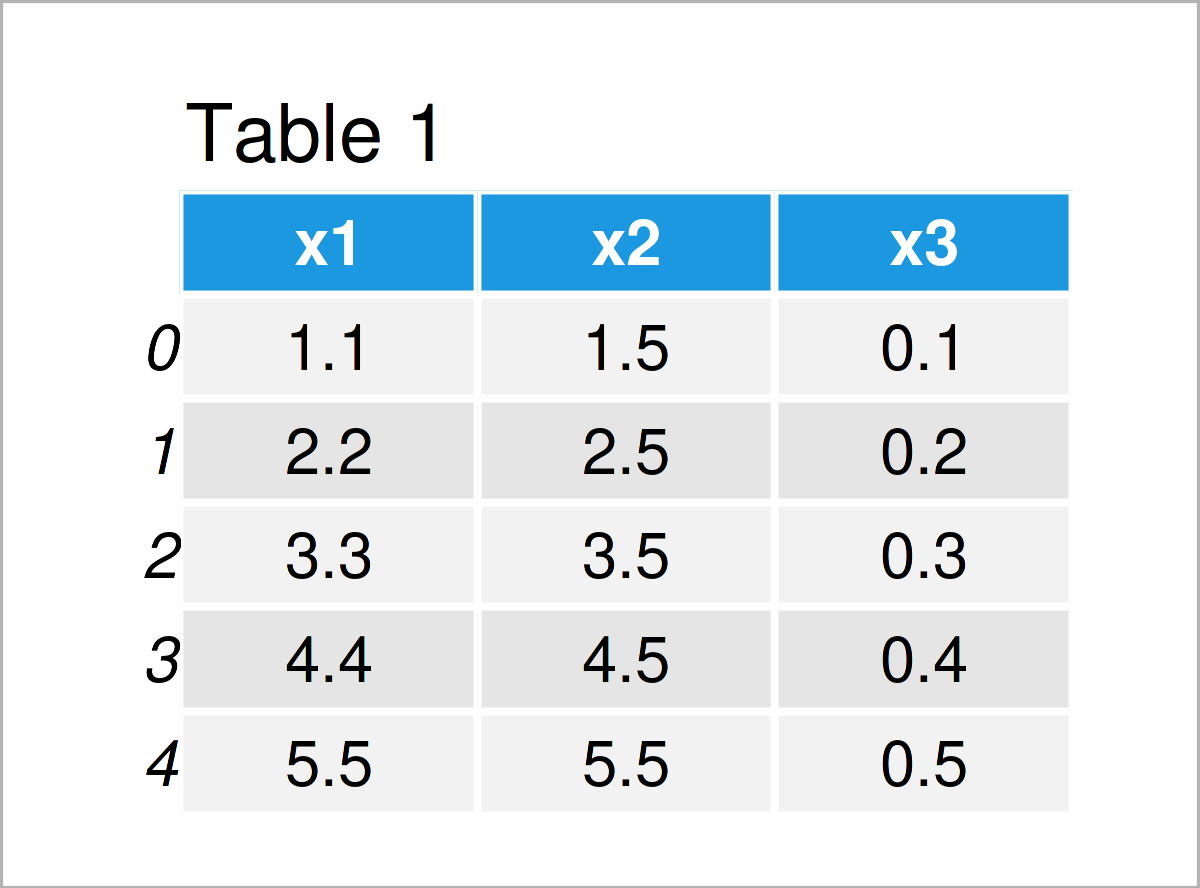# Convert Float to String in pandas DataFrame Column in Python (4 Examples)

This page illustrates how to convert a float column to the string data type in a pandas DataFrame in the Python programming language.

The article will consist of these contents:

Let’s take a look at some Python codes in action…

## Example Data & Libraries

We first have to import the pandas library:

`import pandas as pd                                   # Load pandas`

Let’s also define some example data in Python:

```data = pd.DataFrame({'x1':[1.1, 2.2, 3.3, 4.4, 5.5],  # Create pandas DataFrame
'x2':[1.5, 2.5, 3.5, 4.5, 5.5],
'x3':[0.1, 0.2, 0.3, 0.4, 0.5]})
print(data)                                           # Print pandas DataFrame```Have a look at the previous table. It shows that our example pandas DataFrame is made of five lines and three columns.

Let’s check the data types of the columns in our example DataFrame:

```print(data.dtypes)                                    # Check data types of columns
# x1    float64
# x2    float64
# x3    float64
# dtype: object```

As you can see, all our columns have the float class at this point of the tutorial. Let’s change this!

## Example 1: Convert Single pandas DataFrame Column from Float to String

The following Python programming syntax demonstrates how to convert one single column from the float class to a character string.

To accomplish this task, we can apply the astype function as you can see below:

```data_new1 = data.copy()                               # Create copy of DataFrame
data_new1['x1'] = data_new1['x1'].astype(str)         # Transform float to string```

Let’s have another look at the classes of our pandas DataFrame columns:

```print(data_new1.dtypes)                               # Check data types of columns
# x1     object
# x2    float64
# x3    float64
# dtype: object```

As you can see, the dtype of the first column called x1 has been switched to the object class. Please note that the pandas library stores strings as the object dtype.

## Example 2: Convert Multiple pandas DataFrame Columns from Float to String

The following Python syntax explains how to transform multiple variables of a pandas DataFrame to the string data type in Python.

For this, we can use the astype function once again:

```data_new2 = data.copy()                               # Create copy of DataFrame
data_new2 = data_new2.astype({'x2': str, 'x3': str})  # Transform multiple floats to string```

As in Example 1, we have created a new pandas DataFrame with modified column classes. Let’s print the updated data types:

```print(data_new2.dtypes)                               # Check data types of columns
# x1    float64
# x2     object
# x3     object
# dtype: object```

This time, we have adjusted the data types of the columns x2 and x3. The variable x1 has been kept as in the input DataFrame.

## Example 3: Convert All pandas DataFrame Columns from Float to String

This example illustrates how to convert all columns of a pandas DataFrame from float to the string data type.

To do this, we can apply the astype function as shown below:

```data_new3 = data.copy()                               # Create copy of DataFrame
data_new3 = data_new3.astype(str)                     # Transform all columns to string```

Let’s have another look at the data types of our DataFrame columns:

```print(data_new3.dtypes)                               # Check data types of columns
# x1    object
# x2    object
# x3    object
# dtype: object```

This time, we have created a new data set where all columns have been switched from float to string.

## Example 4: Convert pandas DataFrame Column from Float to String Using apply() Function

In the previous examples, we have used the astype function to convert our float columns to the string data type.

However, it is also possible to use the apply function for this task.

The following Python syntax shows how to use the apply function to parse the data type of the first column (similar to Example 1):

```data_new4 = data.copy()                               # Create copy of DataFrame
data_new4['x1'] = data_new4['x1'].apply(str)          # Transform string to float```

Let’s print the data types of the updated columns:

```print(data_new4.dtypes)                               # Check data types of columns
# x1     object
# x2    float64
# x3    float64
# dtype: object```

As you can see, the data type of the first column has been modified. Please note that the apply function is sometimes considered to be more efficient than the astype function. So if speed is an important metric in your specific situation, you may prefer to use the apply function instead of the astype function.

## Video & Further Resources

I have recently published a video on my YouTube channel, which shows the content of this article. You can find the video below.

Please accept YouTube cookies to play this video. By accepting you will be accessing content from YouTube, a service provided by an external third party.If you accept this notice, your choice will be saved and the page will refresh.

In addition, you might have a look at the related articles which I have published on my website. You can find a selection of articles on related topics such as counting, indices, and groups below.

To summarize: In this tutorial, I have explained how to transform a float variable to the string data type in a pandas DataFrame in Python programming. If you have additional comments or questions, don’t hesitate to let me know in the comments section.

Subscribe to the Statistics Globe Newsletter